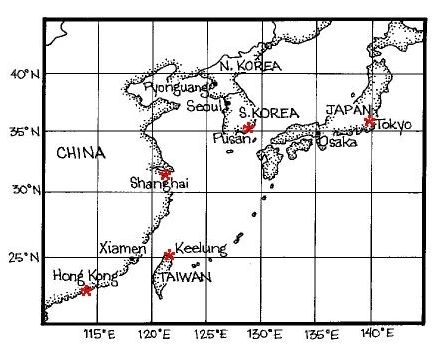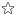quizzes  Forums# Degrees Traveled

created by beckyhoov… on 20 Nov 12, enabled by LouHard level (42% of success)    10 questions - 13 players
How many degrees have you traveled?1
If you started at Shanghai and traveled to Tokyo, about how many degrees of Latitude have you traveled?

2
If you started at Seoul and traveled to Osaka, about how many degrees of Latitude have you traveled?

3
If you started at Hong Kong and traveled to Tokyo, about how many degrees of Latitude have you traveled?

4
If you started at point A and traveled to point B, about how many degrees of Latitude have you traveled?

5
If you started at point A and traveled to point B, about how many degrees of Latitude have you traveled?

6
If you started at point F and traveled to point G, about how many degrees of Latitude have you traveled?

7
If you started at point A and traveled to point E, about how many degrees of Latitude have you traveled?

8
If you started at point E and traveled to point H, about how many degrees of Latitude have you traveled?

9
If you started at point D and traveled to point F, about how many degrees of Latitude have you traveled?

10
If you started at point H and traveled to point G, about how many degrees of Latitude have you traveled?

Quiz.biz does not certify the quizzes to be reliable, contact Beckyhoover !Comment this Quiz
If you want to rank and comment this quizz, login in or create an account !